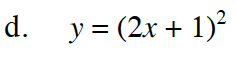### Home > INT2 > Chapter 12 > Lesson 12.1.3 > Problem12-37

12-37.
1. Without graphing, determine if the graph of each equation below has zero, one, or two x‑intercepts. Then describe the roots of each quadratic function. Show all work. Homework Help ✎

2.  a. y = 6x2 + 7x − 20 b. y = x2 − 8x + 16 c. y = 2x2 + x + 3 d. y = (2x + 1)2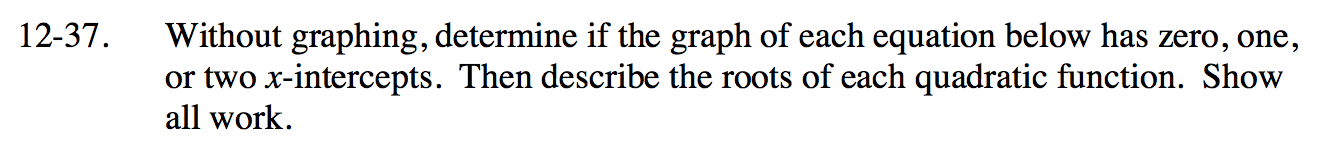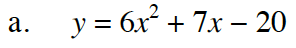0 = (3x − 5)(x + 4)

Two intercepts.

0 = (x − 4)2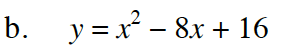The graphs of these equations are all parabolas. To find the x-intercepts, let y = 0 and solve using the Quadratic Formula or factoring and the Zero Product Property.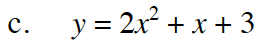No intercepts.